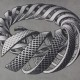# Definition: Natural and Prime Numbers

Here we will elaborate on one of the most important and fundamental mathematical definitions that exists—that of a prime number.  First we will need the definition of a natural number, which I will provide without any explanation or motivation.  Volumes have been written on trying to define natural numbers in an abstract way, but that will do nothing for our discussions here.

Definition 1: A natural number is a positive “whole” number.  It is an element of the set$\{1, 2, 3, 4, 5, 6, ...\}$. //

Notes:

• Zero is not a natural number according to this definition (zero is not positive, it is only non-negative!).
• This set of numbers is infinite—it starts at 1 and goes on forever.
• 1.5, 2.56, and “$\pi$” (the oh-so-famous “Pi”, which is approximately 3.14) are not on this infinite list (only the whole numbers!).

Now let’s do something more interesting.

Definition 2: A prime number is a natural number that is greater than 1 and is only divisible by itself and 1. //

Notes:

• By “divisible” I mean “evenly divisible”, meaning that there is no remainder after dividing.  Thus 4 is divisible by 2 but not by 3, and 10 is divisible by 5 but not by 4.
• 1 is not a prime number, because 1 is not greater than 1!
• 2, 3, 5, 7, 11, 13, and 17 are prime.  For example, 5 ONLY equals$5\times 1$.
• 4, 6, 8, 9, 10, 12 and 14 are not prime.  For example,$4=4 \times 1$ AND$4=2\times 2$, which is not allowed for a prime.
• Every number is either prime or not prime—there is no “in-between” or “both”.  This should be clear, because something is either “divisible by something else” or it isn’t. Period.
• Whole numbers that are not prime are called composite numbers.

Math is not just about making definitions, it is also about proving stuff with these definitions.  Once we’ve made this definition, which we are totally allowed to do, we can now ask questions about the definition.  How many primes are there?  What is the smallest prime?  How many primes are sums of smaller primes?  What patterns exist amongst the primes?

There are an infinite number of questions that we could ask about prime numbers once we have the definition, and some are harder to answer than others.  Elsewhere on this site we have proved that there are an infinite number of primes, but some simple questions about primes have yet to be answered conclusively.  We’ll explain some of these problems later on, but for now just know that there is immense beauty wrapped up in this seemingly harmless definition!

### 2 Responses to Definition: Natural and Prime Numbers

1.Shofy says:

I prefer the uipsde down division way, because you can use it to factor more than one number at once, giving you the GCF and the LCM…the GCF is whatever you can pull out of both, (the stuff on the left side) and the LCM is the GCF multiplied by whatever is leftover at the bottom (the stuff at the left multiplied by the stuff at the bottom–it makes an L shape too). You can also use it to simplify fractions. Your simplified fraction is whatever is leftover at the bottom. Reply

•TrueBeautyOfMath says:

I’m not sure I’m quite understanding what this is in reference to…